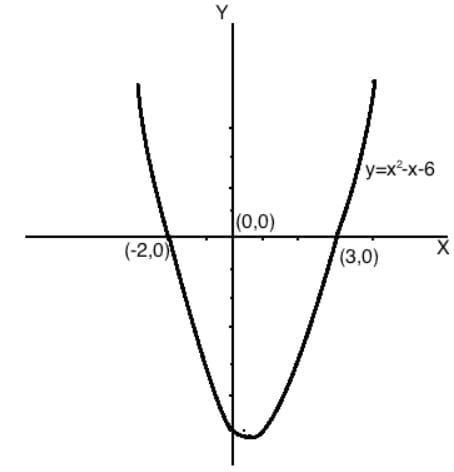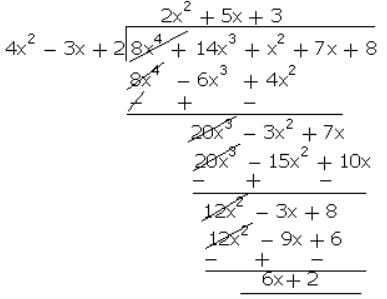Test: Polynomials (Easy)

# Test: Polynomials (Easy) - Class 10

Test Description

## 15 Questions MCQ Test - Test: Polynomials (Easy)

Test: Polynomials (Easy) for Class 10 2023 is part of Class 10 preparation. The Test: Polynomials (Easy) questions and answers have been prepared according to the Class 10 exam syllabus.The Test: Polynomials (Easy) MCQs are made for Class 10 2023 Exam. Find important definitions, questions, notes, meanings, examples, exercises, MCQs and online tests for Test: Polynomials (Easy) below.
Solutions of Test: Polynomials (Easy) questions in English are available as part of our course for Class 10 & Test: Polynomials (Easy) solutions in Hindi for Class 10 course. Download more important topics, notes, lectures and mock test series for Class 10 Exam by signing up for free. Attempt Test: Polynomials (Easy) | 15 questions in 15 minutes | Mock test for Class 10 preparation | Free important questions MCQ to study for Class 10 Exam | Download free PDF with solutions
 1 Crore+ students have signed up on EduRev. Have you?
Test: Polynomials (Easy) - Question 1

### Write down the degree of following polynomials in x: 3 - 2x

Detailed Solution for Test: Polynomials (Easy) - Question 1
The exponent of the first term -2x = 1 Therefore, the degree of the polynomial 3 - 2x = 1

Test: Polynomials (Easy) - Question 2

### Find the degree of the given algebraic expression ax2 + bx + c

Detailed Solution for Test: Polynomials (Easy) - Question 2

We know that the degree is the term with the greatest exponent.

Test: Polynomials (Easy) - Question 3

### If x = 2 and x = 3 are zeroes of the quadratic polynomial x2 + ax + b, the values of a and b respectively are

Detailed Solution for Test: Polynomials (Easy) - Question 3
If 2 and 3 are the zeroes of the quadratic polynomial then (x - 2)(x - 3) are the factors of the polynomial Thus,

Test: Polynomials (Easy) - Question 4

Write the degree of each of the following polynomials: 1 / 2y7 - 12y6 + 48y5 - 10

Detailed Solution for Test: Polynomials (Easy) - Question 4
The degree is the value of the greatest exponent of any expression (except the constant) in the polynomial.

Test: Polynomials (Easy) - Question 5

3x3 - 2x - x + 3 is an example of

Detailed Solution for Test: Polynomials (Easy) - Question 5

3x3 - 2x - x + 3 = 3x3 - 3x + 3 is an example of cubic polynomials. A cubic polynomial is a polynomial of degree 3. Since the highest degree is 3.

Test: Polynomials (Easy) - Question 6

Let p(x) = ax2 + bx + c be a quadratic polynomial. It can have at most

Detailed Solution for Test: Polynomials (Easy) - Question 6

The polynomial ax2 + bx + c has three terms. The first one is ax2, the second is bx, and the third is c. The exponent of the first term is 2. The exponent of the second term is 1 because bx = bx1. The exponent of the third term is 0 because c = cx0. Since the highest exponent is 2, therefore, the degree of ax2 + bx + c is 2. Since, the degree of the polynomial is 2, hence, the polynomial ax2 + bx + c can have zero, one or two zeroes. Hence, the polynomial ax2 + bx + c can have at most two zeros.

Test: Polynomials (Easy) - Question 7

The zero of polynomial p(x) = 4x + 5 is:

Detailed Solution for Test: Polynomials (Easy) - Question 7
Given, f(x) = 4x + 5.

To find the zero of a polynomial, we use f(x) = 0.

Then, 4x + 5 = 0 ⟹ 4x = −5 ⟹ x = -5 / 4

Hence, −5 / 4 ​ is the zero of the polynomial 4x + 5.

Test: Polynomials (Easy) - Question 8

Draw the graphs of y = x2 - x - 6 and find the zeroes in each case.

Detailed Solution for Test: Polynomials (Easy) - Question 8
Clearly graph of y = x2 − x − 6 cut the x-axis at x = −2, 3. ⇒ x = −2, 3 are zeroes of y = x2 − x − 6.

when x = −2; y = 4 + 2 − 6 = 0

when x = 3; y = 9 − 3 − 6 = 0Test: Polynomials (Easy) - Question 9

Assertion: Degree of a zero polynomial is not defined.

Reason: Degree of a non-zero constant polynomial is 0

Detailed Solution for Test: Polynomials (Easy) - Question 9
We know that, The constant polynomial 0 is called a zero polynomial. The degree of a zero polynomial is not defined, a Assertion is true. The degree of a non-zero constant polynomial is zero. A Reason is true. Since both Assertion and Reason are true and Reason is not a correct explanation of Assertion. The correct answer is B.

Test: Polynomials (Easy) - Question 10

Find a quadratic polynomial each with the given numbers as the sum and product of its zeroes respectively: 0, 3

Detailed Solution for Test: Polynomials (Easy) - Question 10
Given: Sum of zeroes = α + β = 0 Product of zeroes = αβ = 3 Then, the quadratic polynomial = x2 - (sum of zeroes )x + product of zeroes = x2 - (0)x + 3 = x2 + 3

Test: Polynomials (Easy) - Question 11

Find the variable of the linear polynomial t + 5.

Detailed Solution for Test: Polynomials (Easy) - Question 11
Since in a polynomial x + a, x is a variable and a is a constant term, therefore, the linear polynomial t + 5 has a variable t and 5 is a constant term.

Test: Polynomials (Easy) - Question 12

Find the zero of the polynomial in each of the following in the following case: p(x) = x +

5

Detailed Solution for Test: Polynomials (Easy) - Question 12
Putting p(x) = 0 we get => x + 5 = 0 => x = - 5

∴ -5 is zero of the polynomial

Test: Polynomials (Easy) - Question 13

The variable in the quadratic polynomial t2 + 4t + 5 is

Detailed Solution for Test: Polynomials (Easy) - Question 13
Since in a standard quadratic polynomial x + x + a, x is a variable and a is a constant term, therefore, in the given polynomial t2 + 4t + 5 we have variable t and 5 is a constant term.

Test: Polynomials (Easy) - Question 14

What must be subtracted from the polynomial 8x4 + 14x3 + x2 + 7x + (8 so that the resulting polynomial is exactly divisible by 4x2 - 3x + 2?

Detailed Solution for Test: Polynomials (Easy) - Question 14Thus, when 6x + 2 is subtracted from the given polynomial 8x4 + 14x3 + x2 + 7x + 8, then it will be divisible by 4x2 - 3x + 2.

Test: Polynomials (Easy) - Question 15

Classify the following polynomial based on their degree: 3x2 + 2x + 1

Detailed Solution for Test: Polynomials (Easy) - Question 15
A polynomial is a function of the form f(x) = anxn + an−1xn−1 +...+ a2x2 + a1x + a0

where an, an−1,... a2, a1, a0. are constants and n is a natural number.

Let p(x) = 3x2 + 2x + 1

The degree of a polynomial is the highest power of x in its expression.

Constant (non-zero) polynomials, linear polynomials, quadratics, cubics and quartics are polynomials of degree 0, 1, 2 , 3 and 4 respectively.

Highest power of x in p(x) is = 2

Thus, the degree of p(x) is 2.

Hence, it is a quadratic polynomial.

Information about Test: Polynomials (Easy) Page
In this test you can find the Exam questions for Test: Polynomials (Easy) solved & explained in the simplest way possible. Besides giving Questions and answers for Test: Polynomials (Easy), EduRev gives you an ample number of Online tests for practice(Scan QR code)